Chemistry Index
Physical Chemistry

Ideal Gas Laws

Boyles Law..... Charles Law..... Equation of State..... Avogradros Law..... Ideal Gas Law..... Daltons Law..... Grahams Law..... Kinetic Theory of Gases.....

Gases

Boyle's Law

When the pressure on a gas is increased at constant temperature the volume decreases.  At constant temperature the volume of a fixed mass of gas is inversely proportional to the pressure.

PV = constant...At constant temperature

P = the pressure
V = volume of fixed mass of gas

Charles Law.. (Gay-Lussac's Law)

The volume of gas at constant pressure increases by the same relative amount for every degree rise in temperature.

Vt   =   Vo + α v.V o.t   =   V o (1 + α v. t) ... At constant pressure
α v= coefficient of expansion (theoretically same for all gases )
Vo = Volume of gas at O o (C or F )
Vt = Volume of gas at t o (C or F )
t = Temperature (Degrees Centigrade /Celcius )

If the temperature is in degrees C then α = approximately 1/273

Vt   =   V o (1 + t /273) ... = At constant pressure

The absolute temperature in degrees Kelvin (K) = the temperature t + 273 Therefore the following relationship holds..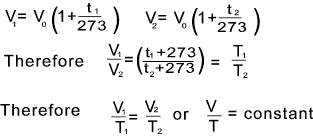These are alternative forms of Gay-Lussac's Law

Equation of State

Gay-Lussac's law and Boyles law can combined to produce an expression which relates pressure, temperature and volume.  This relationship is called the equation of state...   The derivation of the equation of state is as follows;

A gas has a volume V1 at a pressure of P1 and a temperature T1   If the pressure is changed to P2 and the temperature is T2 and the volume resulting is V2.   The relationship is derived as follows:..
a) The temperature is maintained at T1 while the pressure is changed from P1 to P2 The volume is changed from V1 ' according to Boyle's law as follows...

P1V1 = P2V1 ' therefore V1 ' = P1V1 /P2

b) The pressure is now maintained constant and the temperature is changed from T1 to T2 . The volume will then change from V1 ' to V2.  applying Gay-Lussacs' equation.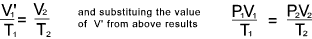The resulting general case of the equation of state for a given mass of gas is...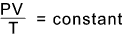Equal volumes of all gases at the same temperature and pressure contain the same number of molecules...

This law simple states that equal numbers of molecules of different gases occupy the same volume at a given temperature and pressure... The constant in the above equation will be independent of the gas providing equal numbers of molecules are involved.  This constant is known as the gas constant (R).   This provides the basis for the general equation of state for any gas..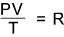the volume V is the volume occupied by one mole i.e the molar volume at the temperature T and pressure P.

Note: A mole is that amount of substance which contains as many elementary particles as there are atoms in 12 gms of carbon 12. (a kmole has as many particles as 12kg of carbon 12 ). The molar mass of (M) of a substance is the mass of 1 mole of the substance.

Ideal Gas Equation..

At a pressure of P and a temperature T the volume of n moles would be n times as great as for 1 mole; if this volume is v the the gas equation becomes...

Pv = nRT

Various units can be used I have chosen the following units

 P = Pressure = (Pa) N/m 2 v = Volume = m 3 T = Absolute Pressure = deg Kelvin R = Universal Gas Constant = 8,314 J /mole.K = 8 314 J /kmole.K n = Number of moles

The laws and rules for ideal gases are only reasonable accurate for gases at low pressures and moderately high temperatures...At pressures around 1 bara or less the ideal gases are generally reasonably accurate for real gases. Example: If we had 1.0 mole of gas at 1.0 atm of pressure at 0oC (273.15 K) (STP), what would be the volume? .....1 Atm = 101 325 Pa. (N/m 2 )

( I use "," as decimal place, "." as multiply symbol, " " for thousands)

(1 . 8,314 . 273,15) / 101 325 = 0.02214 m 3 = 22.14 litres

The molar volume of any ideal gas is 22.14 liters at STP

If this was hydrogen H2 with M = molar mass = 2 grammes then the density = 2/22,14 = 0,09 gm/litre ( kg/m 2)

Dalton's Law of Partial Pressures

This law establishes a connection between the total pressure exerted by the mixture of a number of gases and the pressure exerted by each separate gas.    Dalton's law states .

The partial pressure of each gas in a mixture is defined as the pressure the gas would exert if it alone occupied the whole volume of the mixture at the same temperature.
If a vessel of volume contains two gases n1 moles of gas 1 and n2 moles of gas 2.   If the vessel were only filled with n1 moles of gas 1 the resulting pressure would be p1.   In a similar manner if the vessel were only filled with n2 moles of gas 2 the resulting pressure would be p2.  All the above are considered to be at the same temperature.
The pressure when the two gases are present = P.  Thge law of partial pressures states

P = p1 + p2

This applies to any number of ideal gases...
Developing this relationship further and writing the ideal gas equation for each gas occupying the vessel on its own.

p1 v = n1 RT
p2 v = n2 RT etc..

Hence  (p1 + p2 ) v = (n1 + n2 )RT

Therefore Pv = (n1 +n2 )RT

If n = (n1 + n2 ) = total number of moles in a gas mixture it can be easily proved that

p1 = (n1 / n ).P

If the mole fraction of any gas in a mixture is identified as x a then p a = x a.P

Graham's Law of Diffusion

Diffusion is the tendency for any substance to spread uniformly to fill the space available to it.  This tendency is apparent primarily for gases but it is also exhibited by liquids and solids to much lesser extents...  If two vessels containing to different gases are connected then , whatever the orientation of the vessels the gases will, after a short time peiod each be spread uniformly throughout the jars.  The effects of gravity have very little effect on this process.

It the gas molecules are small enough i.e hydrogen and helium.  The gas may even spread out through the containing vessel wall.  Gases contained in porous earthernware vessel diffuse out into the environment and surrounding gases diffuse in...

When gases diffuse through fine pores and holes the process is often termed "effusion".

Grahams law is another ideal gas law which is only approximate states:

The rate of diffusion (or effusion) of a gas is inversely proportional to the square root of its density

If d1 and d2 are the densities of two gases and M1 and M2 are the associated molecular weights and R1 and R2 are the respective rates of effusion and k is a proportionality constant...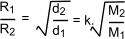Kinetic Theory of Gases

This generally accepted theory provides a close agreement with experimental data of ideal gases under conditions of high temperature and low pressures .  This theory is based on the following postulates.

 A gas is composed of molecules which can be considered as identical, hard smooth spheres The molecules are perfectly elastic with no loss of energy on impacts with other molecules The diameter of the molecules is small compared to the distance moved between impacts, The volume of the molecules is small compared to the volume of the gas The molecules are in complete random motion , impacting with other molecules and the containing walls The time of the impacts is small compared with the time of free motion Attractive and repulsive forces between the molecules are negligible The average kinetic energy of the molecules is proportional to the absolute temperature The pressure generated by the gas results from the impact of the molecules upon the containing walls
Derivations from kinetic Theory

The following notes are in the mks system not the cgs system as is normally used for consideration of molecules...

The derivations illustrated are all approximate but they provide reasonable correct results for ideal gases following the rules relating to the kinetic theory..

Pressure

Consider a cube of sides 'l' aligned in the x, y and z.  The cube is full of "n" molecules each with a mass "m".  The pressure results from the impact of the molecules on the walls of the cube.

Each molecule is moving with a velocity c which has components u,v,w in the x,y and z directions respectively.  c 2 = u 2 + v 2 +w 2
Considering the component of the motion of 1 molecule in the x direction.   The molecule impacts with the wall normal to its direction of motion and rebounds with a velocity -u.   The change of momentum is from mu to -mu = 2mu.  The motion then continues and the molecule hits the opposite wall after a period l/u.   The rate of change of momentum of the molecule is therefore 2mu.u/l = 2mu 2 / l (kg.m/s 2 ).   Likewise rate of change of momentum in the y direction = 2mv 2 /l (kg.m/s 2 and in the z direction is 2mw 2 /l (kg.m/s 2...

Newtons law states that the rate of change of momentum is equal to the force acting.  Therefore the total force due to the impacts of a single molecule =

F = 2 mc 2 / l = 2m (u 2 + v 2 + w 2 ) / l

There are n molecules in the cube each molecule exerting a similar force.   The total force resulting from n molecules is therefore .

Ft = 2 m (c1 2 + c2 2 + c3 2 ...+ cn 2) / l

The mean square velocity c is the average of the squares of the velocities of n molecules is defined as follows.

c = (c1 2 + c2 2 + c3 2 ...+ cn 2) / n

The total force of n molecules is therefore

Ft =2nmc 2 / l (Newtons)

Pressure is defined as the pressure per unit area and the area of the walls of the cube is 6 l 2.  Therefore the resulting pressure =

P =2nmc 2 / (l 6 l 2) = nmc 2 / 3.v .....A

Boyle's Law

J.C. Maxwell (1860) arrived at the conclusion that the mean kinetic energy of the molecules of all gases are the same at constant temperature.

At a constant temperature the kinetic energy mc2 /2 is constant for any gas.   For a fixed mass the number of molecules remains the same and therefore mc2 /2 will be constant.

equation A above can be rewritten as

Pv = nmc 2 / 3 .....Equation B

It is clear from the above notes that at constant temperature the right side of the equation is constant

Pv = Constant

This is in accordance with Boyle's law...

Also following form the equations above

P1v1 = n1m1c1 2 / 3..... and.... P2v2 = n2m2c2 2 / 3

Now if P1 = P2 and v1 = v2 Then

n1m1c1 2 / 3 = n2m2c2 2 / 3

If the gases are at the same temperature then the kinetic energies are the same

m1c12 /2 = m2c22 /2

and therefore

n1 = n2

That is two gases at the same pressure and temperature occupying the same volume contain the same number of molecules.. The agrees with Avogadro's law,

Charles's Law

It is postulated above that the temperature T is proportional to the mean kinetic energy of a gas and therefore from equation B above it follows that

Pv = nkT

where k is a proportionality constant that is is equal

for all gases.  From Maxwells proof that the kinetic energy of all gases is equal at the same temperature it is concluded that k is a universal constant for all gases.  If the pressure is held constant then it is clear from the equation above

v/T = constant

This conclusion agrees the Charles Law (Gay-Lussac's law).... The absolute temperate scale is the same as the temperature defined in reference to the mean kinetic energy of the molecules in an ideal gas

 Relevant Chemistry Sites.. Ideal gas laws - Hyperphysics ...Very good reference for ideal gas laws. Virtual Laboratory for ideal gas law ... An applet allowing the ideal gas law to be tested. Chemi-wiki _Ideal gas laws ...Basic explanation a derivation of the ideal gas laws
Chemistry Index
Physical Chemistry